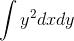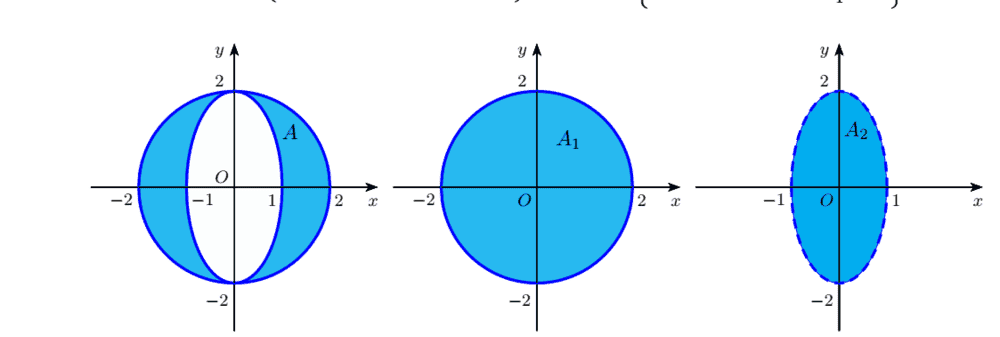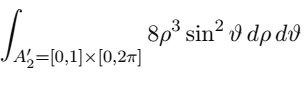# Problem with double integral

• Amaelle
In summary, the problem involves calculating a double integral over a region of integration that consists of two regions. The difficulty lies in converting the integral to polar coordinates and using the appropriate Jacobian. After some calculations, a factor of 2 is obtained from the Jacobian and a further two factors from the integrand, leading to the correct solution.f

#### Amaelle

Homework Statement
integral of int y^2 dxdy over a region (look to the formula inside the exercice)
Relevant Equations
y=rcos(theta)
calculate the double integralover the region of integration is
x^2 + y^2 ≤ 4; x^2 + (y/4)^2 ≥ 1
the integrals have been made over two regionsmy problem is that when I go to the polar coordinate for the ellipsis and use the jacobian i got 2 instead of 8 ( the following is the professor solution)Any help?

$$\int_{A_1}y^2 dx dy = \int_0^{2\pi} d\theta \int_0^2 d\rho\ \rho^3 \sin^2\theta = 16 \int_0^{2\pi} d\theta \int_0^1 d\rho \ \rho^3 \sin^2\theta$$
A half of it,
$$\int_{A_2}y^2 dx dy = 8 \int_0^{2\pi} d\theta \int_0^1 d\rho \ \rho^3 \sin^2\theta=\int_{A_1-A_2}y^2 dx dy$$
What Jacobian you get ?

Last edited:
•Amaelle
Homework Statement:: integral of int y^2 dxdy over a region (look to the formula inside the exercice)
Relevant Equations:: y=rcos(theta)

calculate the double integral
$$\int y^2\,dx\,dy$$over the region of integration is
x^2 + y^2 ≤ 4; x^2 + (y/4)^2 ≥ 1
the integrals have been made over two regions

my problem is that when I go to the polar coordinate for the ellipsis and use the jacobian i got 2 instead of 8 ( the following is the professor solution)

Any help?

For the ellipse, you need to use the parametrization \begin{align*} x &= \rho \cos \vartheta, \\ y &= 2\rho\sin \vartheta. \end{align*} With this choice, the ellipse is at $\rho = 1$, rather than the complicated function of $\vartheta$ you would get from actual polar coordinates.

You should obtain a factor of 2 from the jacobian and a further two factors from the integrand.

•Amaelle
Thanks a lot
so first change
u=x
v=y/2
so the jacobian equal 2
after
v=2rsin(theta)
v^2=4[sin(theta)]^2

I got it now

$$\int_{A_1}y^2 dx dy = \int_0^{2\pi} d\theta \int_0^2 d\rho\ \rho^3 \sin^2\theta = 16 \int_0^{2\pi} d\theta \int_0^1 d\rho \ \rho^3 \sin^2\theta$$
A half of it,
$$\int_{A_2}y^2 dx dy = 8 \int_0^{2\pi} d\theta \int_0^1 d\rho \ \rho^3 \sin^2\theta=\int_{A_1-A_2}y^2 dx dy$$
What Jacobian you get ?
Thanks a lot!